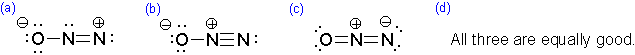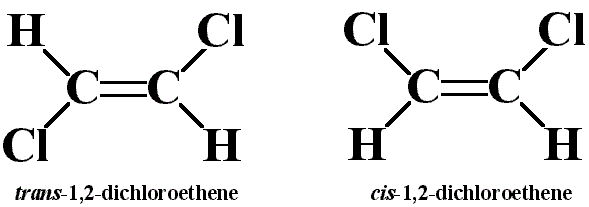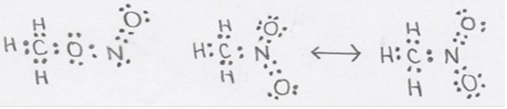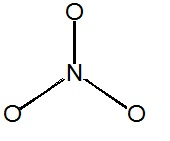# Solution Set 9

$$\newcommand{\vecs}{\overset { \rightharpoonup} {\mathbf{#1}} }$$ $$\newcommand{\vecd}{\overset{-\!-\!\rightharpoonup}{\vphantom{a}\smash {#1}}}$$$$\newcommand{\id}{\mathrm{id}}$$ $$\newcommand{\Span}{\mathrm{span}}$$ $$\newcommand{\kernel}{\mathrm{null}\,}$$ $$\newcommand{\range}{\mathrm{range}\,}$$ $$\newcommand{\RealPart}{\mathrm{Re}}$$ $$\newcommand{\ImaginaryPart}{\mathrm{Im}}$$ $$\newcommand{\Argument}{\mathrm{Arg}}$$ $$\newcommand{\norm}{\| #1 \|}$$ $$\newcommand{\inner}{\langle #1, #2 \rangle}$$ $$\newcommand{\Span}{\mathrm{span}}$$ $$\newcommand{\id}{\mathrm{id}}$$ $$\newcommand{\Span}{\mathrm{span}}$$ $$\newcommand{\kernel}{\mathrm{null}\,}$$ $$\newcommand{\range}{\mathrm{range}\,}$$ $$\newcommand{\RealPart}{\mathrm{Re}}$$ $$\newcommand{\ImaginaryPart}{\mathrm{Im}}$$ $$\newcommand{\Argument}{\mathrm{Arg}}$$ $$\newcommand{\norm}{\| #1 \|}$$ $$\newcommand{\inner}{\langle #1, #2 \rangle}$$ $$\newcommand{\Span}{\mathrm{span}}$$UC Davis CHE 2A: General Chemistry  Instructor: Hayashi Chem 2A        Chem 2B        Chem 2C Reading Assignments Exercises Worksheets Homework Unit I: Atomic Theory            Unit II: Chemical Reactions           Unit III: Gases          Unit IV: Electronic Structure and Bonding

Solutions, hints and answers can be found in the Solutions Page. It is strongly recommended that students do not look at the solutions until after completing or attempting to complete the problems. Going straight to the solutions will reduce the point of the exercises.

### Q1

The central atom in $$BrF_5$$ has how many bonding pairs of electrons and how many non-bonding pairs of electrons.

A. 5 bond pairs, 1 lone pair

### Q2

4. Which of the following molecules does not have a net dipole moment?

1. $$H_2O$$
2. $$NH_3$$
3. $$BF_3$$
4. $$BrF_5$$

A: B

### Q3

What is the bond order in $$NO_2^+$$?

1. 1.5
2. 2
3. 3
4. 4

A: b

### Q4

Which of the following best represents the 3-dimensional view of $$H_2N^-$$?### Q5

Which of the following represents the best resonance structure for $$N_2O$$?A. b

### Q6

Draw acceptable Lewis structures for the following molecules: $$BH_4^-$$, $$NF_3$$, $$CH_3OH$$, $$CO$$, $$H_2CO_3$$ (the hydrogen atoms are bonded to oxygen atoms), $$H_2O_2$$, $$HOF$$, $$H_2CO$$.

### Q7

The remarkable reactivity of fluorine enables the synthesis of an unusually diverse array of compounds of fluorine and iodine. The set includes $$IF$$, $$IF_2^+$$, $$IF_4^+$$, $$IF_4^-$$, $$IF_5$$, and $$IF_8^-$$. Draw the electron-dot (valence-bond) structures of each species and predict its structure.

### Q8

Consider the cis and trans isomers for 1,2-dichloroethene ($$C_2H_2Cl_2$$):Identify the differences in:

1. molecular mass
2. dipole moments
3. Lewis structures
4. molecular geometries​

A. b. trans-1,2-dichloroethene has no dipole moment and is nonpolar, while cis-1,2-dichloroethene has a dipole moment and is polar.

### Q9

Iodine reacts with fluorine to yield several compounds with the molecular formula $$IF_n$$ with $$n$$ varying from 1, 3, 5, and 7.

1. Draw the Lew structure of each.
2. Draw the three-dimensional structure of each molecule with $$n<7$$.

A. The Lewis structures (figure needs lone pairs on fluorines to be indicated)• $$IF$$ ia linear
• $$IF_3$$ is trigonal bipyramidal. The F's and I form a T.
• $$IF_5$$ is square pyramidal with the lone pair is located on the axis of a distorted octahedron.
• Not requested, but $$IF_7$$ does exist and is more advanced than discussed in class. It has 7 equivalent groups of charge are distributed around the iodine atom. Consider a pentagonal bipyramid. 5 F's form a pentagon with the I in the center. Draw an axis through the I and perpendicular to the pentagon. Locate the final two F's on this axis, one above and the other below the pentagon. Another possible structure observed in some cases is the capped octahedron. Construct an octahedron with 6 fluorines at the 6 corners and add the seventh fluorine atom to one of the faces of the octahedron.

### Q10

Draw acceptable Lewis structure(s) for the chlorite anion, $$ClO_2^-$$. What consequences does the Lewis structure(s) have for the bond angle and bond lengths in this anion? What are the bond order(s) of the $$\ce{Cl-O}$$ bonds?

A5An acceptable electron-dot structure should not have formal charge on the chlorine atom. There should be a formal charge of -1 on one of the oxygen atoms such the species is a -1 anion. There are 2 acceptable structures which are equivalent resonance structures. The two Cl-O bonds will have equal bond lengths that will be intermediate in value between a single and double bond. Counting the lone pairs, there are 4 groups of charge around the chlorine so a bent structure is predicted.

### Q11

Two isomers with the stoichiometry $$CH_3NO_2$$ are known: methyl nitrite with the connectivity $$H_3CONO$$ and nitromethane with the connectivity $$H_3CNO_2$$.

1. Draw the Lewis structures of the two isomers. Include resonance structures as appropriate. Provide the formal charges of the heavy, i.e. non-hydrogen, atoms.
2. Compare the nitrogen-oxygen bond lengths of the two isomers. Discuss the basis for your order.
3. Predict the $$CON$$ and $$ONO$$ bond angles in methyl nitrite.
4. In which isomer would you expect all the heavy atoms to lie in the same plane?

CH3ONO, C & O adjacent to C, sp3;N & other O, sp2; formal charge on all atoms is 0.

CH3NO2, C, sp3; all others, sp2; formal charge: 0 on C and doubly bonded O, +1 on N and -1 on the singly bonded O.b) Compare the nitrogen-oxygen bond lengths of the two isomers. Discuss the basis for your order.

• One electron-dot structure is possible for methyl nitrite with a N,O single bond and a N,O double bond. The former is shorter. We note two resonance structures in the case of nitromethane so the N,O bond length is halfway between a single and double bond. rN=O(methyl nitrite) < rN,O(nitromethane) < rN-O(methylnitrite)

c) Predict the CON and ONO bond angles in methyl nitrite.

• There are 4 groups of charge around the O connected by single bonds to the N and C. Don't overlook the lone pairs! Therefore tetrahedral geometry with ÐCON » 109°. In contrast, there are 3 groups of charge around the nitrogen so trigonal planar geometry with ÐONO » 120° is expected.

d) In which isomer would you expect all the heavy atoms to lie in the same plane?

Nitromethane is a sure winner. The oxygen atoms and the carbon atom are directly attached to the nitrogen atom which has 3 groups of charge around it and therefore a trigonal planar geometry. All 4 atoms must therefore lie in the same plane.

### Q12

Fill in the following tables (follows octet rules):

 Species # of electron groups VB hybridization # of atoms bonded to central atom electronic geometry name molecular geometry name bond angles Polar or nonpolar $$CS_2$$ 2 sp 2 linear linear 180° mon-polar $$SO_3$$ 3 sp2 3 trigonal planar trigonal planar 120° non-polar $$SO_3^{2-}$$ 4 sp3 3 tetrahedral trigonal pyramidal 109.5° polar $$NH_3$$ 4 sp3 3 tetrahedral trigonal pyramidal 109.5° polar $$SF_6$$ 6 sp3d2 6 octahedral octahedral 90°, 180° non-polar $$O_3$$ 3 sp2 2 trigonal planar bent 120° polar $$SF_4$$ 5 sp3d 4 trigonal bipyramidal seesaw 90°, 120°, 180° polar $$XeF_4$$ 6 sp3d2 4 octahedral square planar 90°, 180° non-polar $$PO_4^{3-}$$ 4 sp3 4 tetrahedral tetrahedral 109.5° non-polar $$CH_2Cl_2$$ 4 sp3 4 tetrahedral tetrahedral 109.5° polar

## Q13

Use the information in the table of Q12 to draw each of the (3-D) molecules.

### Q14

Write resonance structures for the nitrate ion ($$NO_3^-$$), if any exist.

There are

$5+(3\times6)+1=24$

valence electrons. The Lewis structure shows that structures can be drawn with the electrons delocalized over multiple oxygen pairs.Notice that the nitrogen atom does not have a proper octet, so the a pair of electrons must be taken from an oxygen atom to form a new double bond (you can pick from any of the three oxygen atoms).As with all resonance structure, none of the above structures is the true structure, which is an average of all three, and cannot be represented by a single Lewis structure.

Each oxygen/nitrogen bond is stronger than a simple single bond. Take the average of the bond order for the resonance structures to ge the "accurate" bond order. For example, the top nitrogen/oxygen bond will have a bond order of

$\dfrac{1 + 2 + 1}{3} = \dfrac{4}{3}$

By symmetry, each of the other two bonds will have the same bond order.

NOTE: In the $$NO_3^-$$ ion, each resonance structure are equivalent and therefore will have the same weight in determining the nature of the electronic structure. This is why the bond order was calculated as a simple average above. However, this is not the case with all molecules and in fact some resonance structures may be nonequvalent and will carry greater weights and more closely resembles the true electronic structure of the molecule/ion. However, this is not an aspect typically discussed in general chemistry classes.

Solution Set 9 is shared under a not declared license and was authored, remixed, and/or curated by LibreTexts.